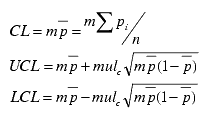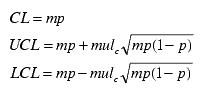# Np chart calculations

Np chart control limits are calculated as follows:

 Control Limits calculated from data Control Limits calculated from standard (mean of fraction of nonconformity ( p ) is given)## Descriptive Statistics

• Lower Control Limit (LCL)

• Upper Control Limit (UCL)

• Center Line (CL)